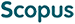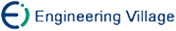黄成泉, 王士同, 蒋亦樟. 熵指数约束的模糊聚类新算法[J]. 计算机研究与发展, 2014, 51(9): 2117-2129.
 引用本文: 黄成泉, 王士同, 蒋亦樟. 熵指数约束的模糊聚类新算法[J]. 计算机研究与发展, 2014, 51(9): 2117-2129.Huang Chengquan, Wang Shitong, Jiang Yizhang. A New Fuzzy Clustering Algorithm with Entropy Index Constraint[J]. Journal of Computer Research and Development, 2014, 51(9): 2117-2129.
 Citation: Huang Chengquan, Wang Shitong, Jiang Yizhang. A New Fuzzy Clustering Algorithm with Entropy Index Constraint[J]. Journal of Computer Research and Development, 2014, 51(9): 2117-2129.## A New Fuzzy Clustering Algorithm with Entropy Index Constraint

• 摘要: 针对基于模糊C均值聚类(fuzzy C-means, FCM)算法框架的竞争聚集聚类(competitive agglomeration, CA)算法中模糊指数m被限定为2的问题,提出了一种更为普适的模糊聚类新算法.该算法首先在FCM算法框架的基础上引入熵指数约束条件，构造了基于熵指数约束的模糊C均值聚类(entropy index constraint FCM, EIC-FCM)算法，成功地将模糊指数m>1的约束条件转换为熵指数0

Abstract: The fuzziness index m plays an important role in the clustering result of fuzzy clustering algorithms. In order to avoid the fuzziness index m of the CA (competitive agglomeration) clustering algorithm based on FCM (fuzzy C-means) clustering algorithm framework being forced to fix at the usual value 2, a more universal fuzzy clustering algorithm is proposed. Firstly, a fuzzy clustering algorithm named EIC-FCM (entropy index constraint FCM), which has comparable clustering performance to the classical FCM algorithm, is presented by introducing an entropy index r into constraints with m=1. The successful introducing of entropy index r effectively makes the fuzziness index constraint m>1 transform into entropy index constraint 0/下载:  全尺寸图片 幻灯片
• 分享
• 用微信扫码二维码

分享至好友和朋友圈